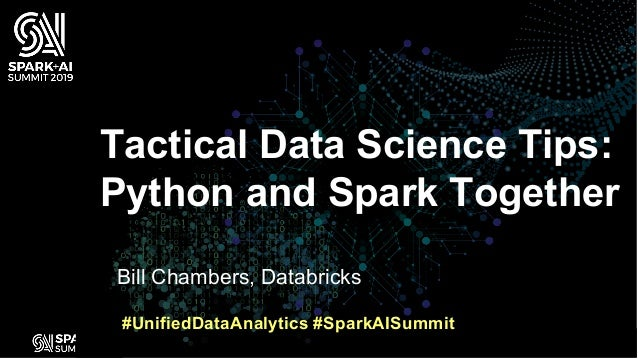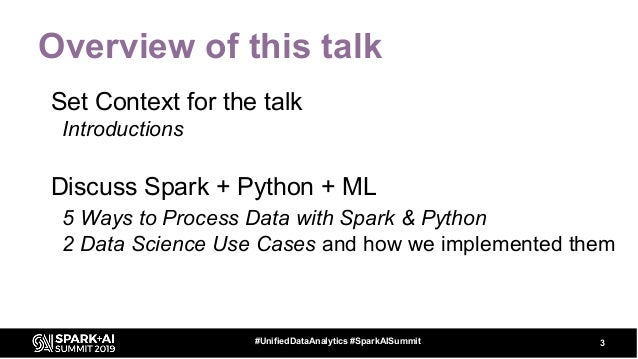Successfully reported this slideshow.Upcoming SlideShare
×

ofUpcoming SlideShare
Next

0 Likes

Share

# Tactical Data Science Tips: Python and Spark Together

Running Spark and Python data science workloads can be challenging given the complexity of the various data science tools in the ecosystem like sci-kit Learn, TensorFlow, Spark, Pandas, and MLlib. All these various tools and architectures, provide important trade-offs to consider when it comes to moving to proofs of concept and going to production. While proof of concepts may be relatively straightforward, moving to production can be challenging because it’s difficult to understand not just the short term effort to develop a solution, but the long term cost of supporting projects over the long term.

This talk will discuss important tactical patterns for evaluating projects, running proofs of concept to inform going to production, and finally the key tactics we use internally at Databricks to take data and machine learning projects into production. This session will cover some architectural choices involving Spark, PySpark, Pandas, notebooks, various machine learning toolkits, as well as frameworks and technologies necessary to support them.

### Related Books

#### Free with a 30 day trial from Scribd

See all
• Be the first to like this

### Tactical Data Science Tips: Python and Spark Together

1. 1. WIFI SSID:Spark+AISummit | Password: UnifiedDataAnalytics
2. 2. Bill Chambers, Databricks Tactical Data Science Tips: Python and Spark Together #UnifiedDataAnalytics #SparkAISummit
3. 3. Overview of this talk Set Context for the talk Introductions Discuss Spark + Python + ML 5 Ways to Process Data with Spark & Python 2 Data Science Use Cases and how we implemented them 3#UnifiedDataAnalytics #SparkAISummit
4. 4. Setting Context: You Data Scientists vs Data Engineers? Years with Spark? 1/3/5+ Number of Spark Summits? 1/3/5+ Understanding of catalyst optimizer? Yes/No Years with pandas? 1/3/5+ Models/Data Science use cases in production? 1/10/100+ 4#UnifiedDataAnalytics #SparkAISummit
5. 5. Setting Context: Me 4 years at Databricks ~0.5 yr as Solutions Architect, 2.5 in Product, 1 in Data Science Wrote a book on Spark Master’s in Information Systems History undergrad 5#UnifiedDataAnalytics #SparkAISummit
6. 6. Setting Context: My Biases Spark is an awesome(but a sometimes complex) tool Information organization is a key to success Bias for practicality and action 6#UnifiedDataAnalytics #SparkAISummit
7. 7. 5 ways of processing data with Spark and Python
8. 8. 5 Ways of Processing with Python RDDs DataFrames Koalas UDFs pandasUDFs 8#UnifiedDataAnalytics #SparkAISummit
9. 9. Resilient Distributed Datasets (RDDs) rdd = sc.parallelize(range(1000), 5) rdd.map(lambda x: (x, x * 10)).take(10) [0, 1, 2, 3, 4, 5, 6, 7, 8, 9] [(0, 0), (1, 10), (2, 20), (3, 30), (4, 40), (5, 50), (6, 60), (7, 70), (8, 80), (9, 90)] 9#UnifiedDataAnalytics #SparkAISummit
10. 10. Resilient Distributed Datasets (RDDs) What that requires… 10#UnifiedDataAnalytics #SparkAISummit JVM Serialize row Python Process Deserialize Row Perform Operation Python Process Serialize Row deserialize row JVM
11. 11. Key Points Expensive to operate (starting and shutting down processes, pickle serialization) Majority of operations can be performed using DataFrames (next processing method) Don’t use RDDs in Python 11#UnifiedDataAnalytics #SparkAISummit
12. 12. DataFrames 12#UnifiedDataAnalytics #SparkAISummit df = spark.range(1000) print(df.limit(10).collect()) df = df.withColumn("col2", df.id * 10) print(df.limit(10).collect()) [Row(id=0), Row(id=1), Row(id=2), Row(id=3), Row(id=4), Row(id=5), Row(id=6), Row(id=7), Row(id=8), Row(id=9)] [Row(id=0, col2=0), Row(id=1, col2=10), Row(id=2, col2=20), Row(id=3, col2=30), Row(id=4, col2=40), Row(id=5, col2=50), Row(id=6, col2=60), Row(id=7, col2=70), Row(id=8, col2=80), Row(id=9, col2=90)]
13. 13. DataFrames 13#UnifiedDataAnalytics #SparkAISummit df = spark.range(1000) print(df.limit(10).collect()) df = df.withColumn("col2", df.id * 10) print(df.limit(10).collect()) [Row(id=0), Row(id=1), Row(id=2), Row(id=3), Row(id=4), Row(id=5), Row(id=6), Row(id=7), Row(id=8), Row(id=9)] [Row(id=0, col2=0), Row(id=1, col2=10), Row(id=2, col2=20), Row(id=3, col2=30), Row(id=4, col2=40), Row(id=5, col2=50), Row(id=6, col2=60), Row(id=7, col2=70), Row(id=8, col2=80), Row(id=9, col2=90)]
14. 14. DataFrames What that requires… 14#UnifiedDataAnalytics #SparkAISummit Spark’s Catalyst Internal Row Spark’s Catalyst Internal Row
15. 15. Key Points Provides numerous operations (nearly anything you’d find in SQL) By using an internal format, DataFrames give Python the same performance profile as Scala 15#UnifiedDataAnalytics #SparkAISummit
16. 16. Koalas DataFrames 16#UnifiedDataAnalytics #SparkAISummit import databricks.koalas as ks kdf = ks.DataFrame(spark.range(1000)) kdf['col2'] = kdf.id * 10 # note pandas syntax kdf.head(10) # returns pandas dataframe
17. 17. Koalas: Background Use Koalas - a library that aims to make the pandas API available on Spark. pip install koalas pandaspyspark
18. 18. Koalas DataFrames What that requires… 18#UnifiedDataAnalytics #SparkAISummit Spark’s Catalyst Internal Row Spark’s Catalyst Internal Row
19. 19. Key Points Koalas gives some API consistency gains between pyspark + pandas It’s never go to match either pandas or PySpark fully Try it and see if it covers your use case, if not move to DataFrames 19#UnifiedDataAnalytics #SparkAISummit
20. 20. Key gap between RDDs + DataFrames No way to run “custom” code on a row or a subset of the data Next two transforming methods are “user-defined functions” or “UDFs” 20#UnifiedDataAnalytics #SparkAISummit
21. 21. DataFrame UDFs df = spark.range(1000) from pyspark.sql.functions import udf @udf def regularPyUDF(value): return value * 10 df = df.withColumn("col3_udf_", regularPyUDF(df.col2)) 21#UnifiedDataAnalytics #SparkAISummit
22. 22. DataFrame UDFs What it requires… 22#UnifiedDataAnalytics #SparkAISummit JVM Serialize row Python Process Deserialize Row Perform Operation Python Process Serialize Row deserialize row JVM
23. 23. Key Points Legacy Python UDFs are essentially the same as RDDs Suffer from nearly all the same inadequacies Should never be used in place of PandasUDFs (next processing method) 23#UnifiedDataAnalytics #SparkAISummit
24. 24. DataFrames + PandasUDFs df = spark.range(1000) from pyspark.sql.functions import pandas_udf, PandasUDFType @pandas_udf('integer', PandasUDFType.SCALAR) def pandasPyUDF(pandas_series): return pandas_series.multiply(10) # the above could also be a pandas DataFrame # if multiple rows df = df.withColumn("col3_pandas_udf_", pandasPyUDF(df.col2)) 24#UnifiedDataAnalytics #SparkAISummit
25. 25. DataFrames + PandasUDFs 25#UnifiedDataAnalytics #SparkAISummit JVM Serialize Catalyst to Arrow deserialize arrow as pandas DF or Series Perform Operation Serialize to arrow deserialize to Catalyst Format JVM Optimized with pandas + NumPyOptimized with Apache Arrow
26. 26. Key Points Performance won’t match pure DataFrames Extremely flexible + follows best practices (pandas) Limitations: Going to be challenging when working with GPUs + Deep Learning Frameworks (connecting to hardware = challenge) Batch size to pandas must fit in memory of executor 26#UnifiedDataAnalytics #SparkAISummit
27. 27. Conclusion from 5 ways of processing Use Koalas if it works for you, but Spark DataFrames are the “safest” option Use pandasUDFs for user-defined functions 27#UnifiedDataAnalytics #SparkAISummit
28. 28. 2 Data Science use cases and how we implemented them
29. 29. 2 Data Science Use Cases Growth Forecasting 2 methods for implementation: a. Use information about a single customer – Low n, low k, high m b. Use information about all customers – High n, low k, low m Churn Prediction Low n, low k, low m 29
30. 30. 3 Key Dimensions How many input rows do you have? [n] Large (10M) vs small (10K) How many input features do you have? [k] Large (1M) vs small (100) How many models do you need to produce? [m] Large (1K) vs small (1) 30#UnifiedDataAnalytics #SparkAISummit
31. 31. Growth Forecasting (variation a) (low n, low k, high m) “Historical” Approach: • Collect to driver • Use RDD pipe to try and send it to another process or other RDD code 31 “Our” Approach: • pandasUDF for distributed training (of small models)
32. 32. Growth Forecasting (variation b) (high n, low k, low m) “Historical” Approach: • Use Spark’s MLlib; distributed algorithms to approach the problem 32 Our Approach: • MLlib • Horovod
33. 33. Churn Prediction (low n, low k, low m) “Historical” Approach: • Tune on a single machine • Complex process to distribute training 33 Our Approach: • pandasUDF for distributed hyper- parameter tuning (of small models)
34. 34. Code walkthrough of each method Code gist.github.com/anabranch for code 34
35. 35. The Final Gap Each of these methods operate slightly differently Some distributed, some not Consistency in production is essential to success. Know input and outputs, features, etc. 35
36. 36. MLFlow is key to our success Allows tracking of all inputs to model + results - Inputs (data + hyperparameters) - Models (trained and untrained) - Rebuild everything after the fact Code gist.github.com/anabranch for code 36
37. 37. DON’T FORGET TO RATE AND REVIEW THE SESSIONS SEARCH SPARK + AI SUMMIT

Total views

660

On Slideshare

0

From embeds

0

Number of embeds

0

24

Shares

0